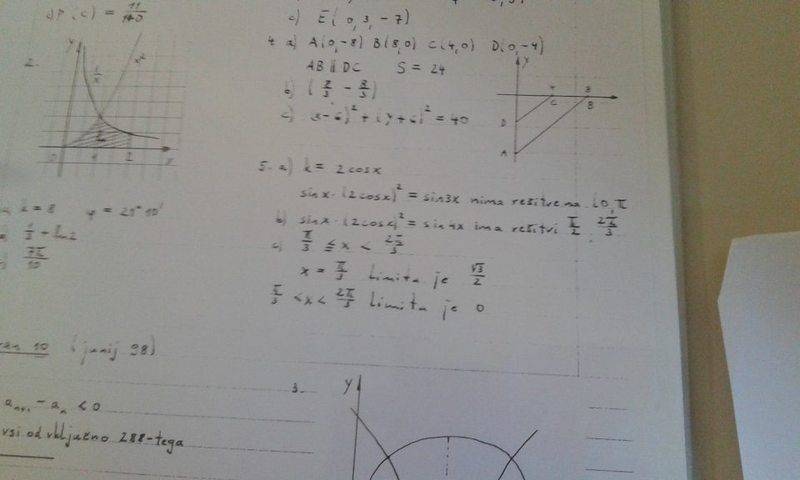# Find the limit of a sequence

## Homework Statement

given a geometric sequence sin(x),sin(2x), . . .
c) find for which values of x∈(0,π) this sequence converges and calculate its limit

|q|<1 or -1<q<1

## The Attempt at a Solution

Ok so in part a) and b) i calculated the quotient and found out that
q=sin2x/sinx
q=(2*cos(x)*sin(x))/(sin(x))
q=2*cos(x)
so know i tried to figure out for which values x will converge
|q|<1
-1<q<1
-1<2*cos(x)<1 /:2
-1/2<cos(x)<1/2
2π/3>x>π/3
which means that the sequence will converge when the angle is anywhere between 120° and 60°
However i'm unsure now how to continue to find the limit because I've never seen an example where the value x isin't exactly defined
could someone show me how this is done or give me some kind of a hit or check if I made a mistake somewhere inbetween
Thanks

Last edited:

PeroK
Homework Helper
Gold Member
2021 Award
What makes you think that is s geometric sequence?

Also, you use both the terms series and sequence. Which is it?

•Matejxx1
I'm really sorry I made a mistake while translating the text into english.
Also it is written in the problem that this is a geometric sequence

HallsofIvy
Homework Helper
And the sequence is sin(x), 2 cos(x)sin(x), 4cos^2(x)sin(x), 8 cos^3(x)sin(x), ... ?
Yes, the "geometric sequence a, ar, ar^2, ar^3, ... converges for -1< r< 1 and converges to a/(1- r). Here you are saying that a= sin(x) and r= 2cos(x).

•Matejxx1
PeroK
Homework Helper
Gold Member
2021 Award
I'm really sorry I made a mistake while translating the text into english.
Also it is written in the problem that this is a geometric sequence
Perhaps there is a problem in translation, but clearly ##sin(nx)## is not in general a geometric sequence. Take ##x = \pi/2## for example.

•Matejxx1
Perhaps it will be better if I write the whole problem out not just the last step I'll try to do that right now

And the sequence is sin(x), 2 cos(x)sin(x), 4cos^2(x)sin(x), 8 cos^3(x)sin(x), ... ?
yes that's the sequence.
converges to a/(1- r). Here you are saying that a= sin(x) and r= 2cos(x).
but woudn't that just give me the sum of all elements of the sequence?
Edit :
I asked my profesor if he could give me the solutions and he didn't have much time but I did get to take a picture if this helps in any wayso the solutions to 5 c)
are π/3 ≤ x < 2*π/3 --------- it is the same that I got but I don't know why there is an equal sign?
x=π/3 ----------- the limit is sqrt(3)/2
π/3 < x < 2*π/3 the limit is 0 <------------------- i get this because if -1< r< 1 the sequence converges to 0

PeroK
Homework Helper
Gold Member
2021 Award
So, the question is: For which values of x does the geometric sequence converge:

##sinx, sinx(2cosx), sinx(2cosx)^2 \dots##

•Matejxx1
yes basically

PeroK
Homework Helper
Gold Member
2021 Award
yes basically
Why do you think ##|q| < 1##?

•Matejxx1
Well, I though if the series converges the sequence would converge as well.
Therefore I went ahead and wrote that |q|<1

PeroK
Homework Helper
Gold Member
2021 Award
Many sequences converge when the corresponding series does not.

•Matejxx1
Hmmm, now that you pointed it out if q=1 the limit of the sequence would simply be sin(x)

Ray Vickson
Homework Helper
Dearly Missed

## Homework Statement

given a geometric sequence sin(x),sin(2x), . . .
c) find for which values of x∈(0,π) this sequence converges and calculate its limit

Is the sequence
sin(x), sin(2x), sin(3x), sin(4x), sin(5x), ...
or is it
sin(x), sin(x), sin(4x), sin(8x), sin(16x), ....?

•Matejxx1
the sequence is
sin(x), sin(x)*2cos(x), sin(x)*(2*cos(x))2, sin(x)*(2*cos(x))3, sin(x)*(2*cos(x))4, . . .

Many sequences converge when the corresponding series does not.

i think I get it now
the sequence
an=sin(x)*(2*cos(x))n-1 will converge when either |2*cos(x)|<1--------- when that is true the sequence will converge to 0
or when 2*cos(x)=1
2*cos(x)>1 this is not possbile because a limit would not exist here
2*cos(x)=-1 because the limit doesnt exist here either

PeroK
Homework Helper
Gold Member
2021 Award
i think I get it now
the sequence
an=sin(x)*(2*cos(x))n-1 will converge when either |2*cos(x)|<1--------- when that is true the sequence will converge to 0
or when 2*cos(x)=1
2*cos(x)>1 this is not possbile because a limit would not exist here
2*cos(x)=-1 because the limit doesnt exist here either